This article discusses the wave propagation in graded-index fibers. We will calculate the number of propagable waves and the optimal shape of the refractive index profile.

Single Mode Fibers

Single mode fibers have a high bandwidth, but due to the generally small fiber parameter V, they either have a small core diameter or a small numerical aperture (N.A.). This makes the coupling between fibers and devices hard.

Step-Index Multi-Mode Fibers

On the other hand, multi-mode step-index fibers (high V) the eigenmodes have in general different propagation delays, resulting in a pulse broadening calculated below: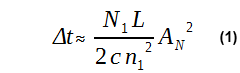N1 is the group index in the fiber core and L is the fiber length. This limits the transmission rate to about 20 to 100Mbit/s on a 1km fiber length.

So graded-index fiber was invented so that all the eigenmodes have nearly the same propagation time.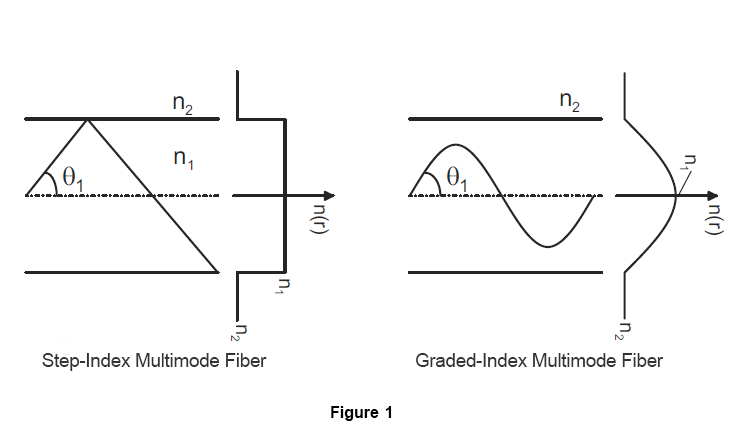### 1. Field Calculation

For linearly polarized waves in graded-index fibers the scalar wave equation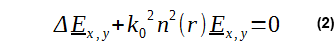is effective. Firstly an x-direction linearly polarized wave of the formis assumed. Therefore the scalar wave equation for ψ(r,φ) results in: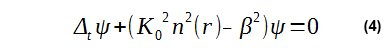in cylindrical coordinate this yields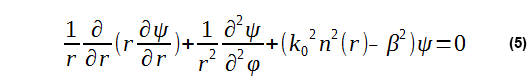In contrast to step-index fibers, the refractive index n(r) is now dependent on the radial coordinate r. For a LPlp-wave with the circumferential order l for ψ(r,φ) the result is: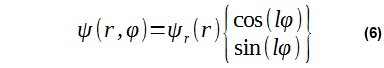Inserting this approach into the wave equation (5), the radial field variable ψr(r) can be obtained:Therefore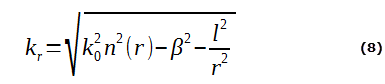can be interpreted as wave number in radial direction (see Figure 2) below. The zeros of kr2 are r1 and r2, which are called the inner and outer turning radius.

kr    -is real for r1 < r < r2                           ====>  ψ oscillating

kr    -is imaginary for r < r1  or r > r2         ====>  ψ decays exponentially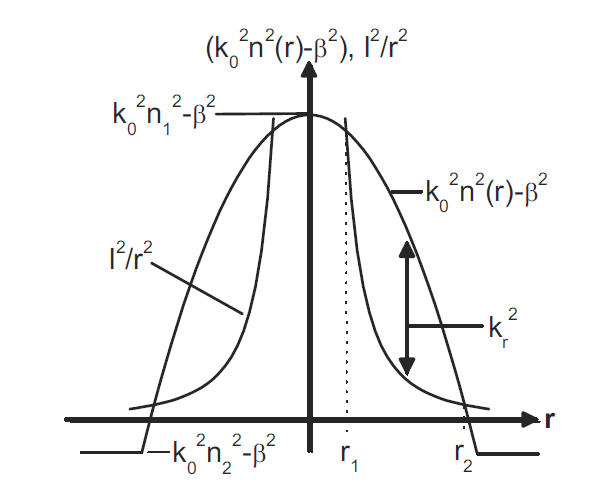Figure 2: Wave number in radial direction kr and the turning radius r1 and r2

Thus, the field is concentrated in the region between r1 and r2.

In order to avoid the singularities at r = 0 in equation (7), the substitution r = a exp(ω) is applied. Then the wave equation is given by: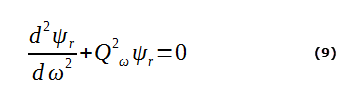with Qω2 = rkr2.

It is Qω2 = 0 for ω1 and ω2. Thus r1 = a exp(ω1) and r2 = a exp(ω2) apply.

as long as |Qω| is sufficiently large and is only weakly dependent of ω (r respectively), equation (9) is approximately solved by the WKB-solution (Wentzel, Kramers, Brillouin):As long as Qω ≠ 0 applies, three cases apply.

1.  ω < ω1  ==> Qω2, kr2 < 0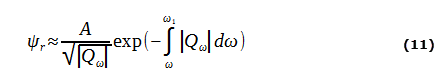2. ω1 < ω < ω2   ==>  Qω2, kr2 > 0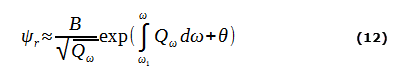3. ω > ω2   ==>  Qω2, kr2 < 0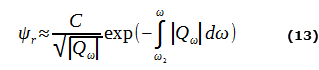where A, B and C in (11), (12), (13) designate constants.Figure 3: WKB-solution for problem (a) with one turning point and (b) with two turning points.

In order for (11) and (12), as well as (12) and (13) to be continuously differentiale at ω ≈ ω1, and ω ≈ ω2, respectively, it follows from Figure 3, that for ω --> ω1 the argument of the cosine in equation (12) must take the value -π/4 and for ω --> ω2 the value mπ+π/4 (m - of integer).

These intuitive considerations are confirmed by accurate calculations. In addition, A = C = B/2.

Therefore the result is given bywith the radial modal number p = 1,2,3... of the LPlp-wave.

With the relation dr = r dω, (14) can be determined as: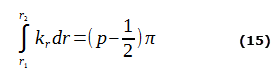Because of the approximation in equation (10), the equation (14) and (15) only apply for p >> 1.

For particular LPlp-waves the propagation constant β can be determined from (15). If l and p are given, β has to be chosen to satisfy (15) (in general, only numerically feasible).Figure 4: Field representation of the  LPlp-wavesFigure 5:
(a) Spiral-shaped beam path in a graded-index fiber with a parabolic refractive index profile
(b) Cross-section projection of a beam path

### 2. Number of Propagable Modes

For modal number combination (l,p) there are:

2 modes for l = 0 (2 polarizations)

4 modes for l ≠ 0 (2 polarizations and 2 orientations, sin(l,φ) and cos(l,φ))

A refractive index profile n(r) is given, that declines monotonously for a rising r (r<a) and that remains constant n(r) = n2 for r ≥ a. To answer the question of number of guided modes and therefore the modal number of combinations(l,p), equation (15) is evaluated. Equation (8) and (15) result in: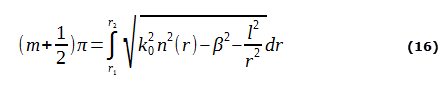with the radial modal numbers m = (p-1) (m = 0, 1, 2, ...).

Assuming a waveguide with large number of guided modes, equation (16) can be approached by: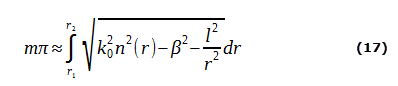The maximum radial modal or order number m = mmax is reached for β = k0n2 with a given l. Thus:In this equation r1 and r2 are the turning radii for β = k0n2. Then the total number of modes M can be calculated by: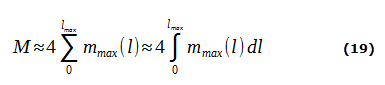The factor 4 follows from the four possible modes for a given modal number combination (l,p), where l ≠ 0. Inserting mmax from equation (18), therefore: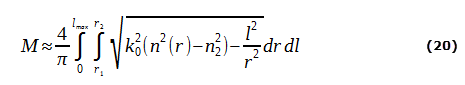In equation (20) the integration proceed firstly over r and afterwards over l (Integration limits shown in Figure 6 below).Figure 6: Representation of the integration limits in the r, l-plane

The order is reversed to simplify the integration: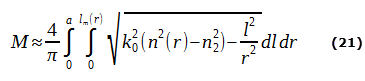The radial order lm(r) is given by the fact, that for l = lm(r) the radial wave number results in kr2 = k02(n2(r) - n22) - l2/r2 = 0.

Therefore: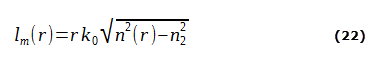Thus, after the integration over l: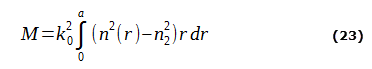The number of guided modes is proportional to the rotation volume, which is formed by the refractive index profile.

#### Examples

1. Example: parabolic refractive index profileΔ represents the relative refractive number difference: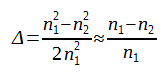In this case, the number of modes is given by:with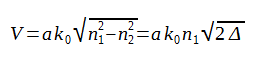2. Example: Step-index fiber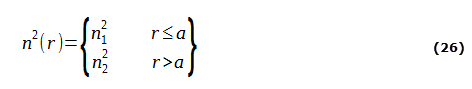In this case the number of modes is:For a typical multi-mode optical fiber with 2a = 50µm, n1 = 1.45, Δ = 0.01, AN = 0.205 and λ = 1.3µm there are approximately 152 modes guided in a parabolic refractive profile and 306 propagable modes for a step-index profile.

### 3. Propagation delay between the eigenmodes

#### Profile Dispersion

The aim of the following calculations is to determine a refractive index profile in which all the modes have almost the same propagation delay. According to the optical beam model, the path of a beam belonging to a wave is determined through the refractive index profile n(r) and the propagation delay of the wave along the beam path is described by the group index N(r). Thus the delay depends obviously on both the refractive index profile n(r) and the group index N(r).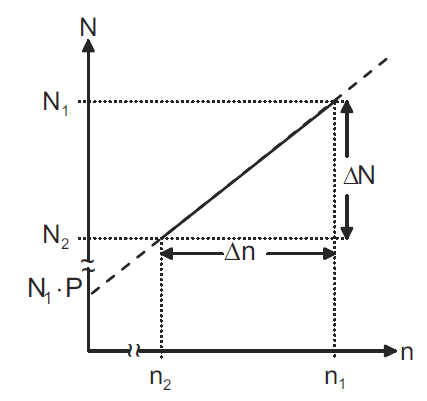Figure 7: Group index N(r) as a function of the refractive index n(r)

A linear relation between n(r) and N(r) is assumed by Figure 7 above.

For example this relation can be described as:The parameter P is called the profile dispersion parameter.  If equation (28) is true, the parameter is called linear profile dispersion. Thus, P depends only on the wavelength λ and not on the radius r.

In order to explain the term of the profile dispersion, the relation between Δn and ΔN in figure (7) will be analyzed in details. The refractive index difference Δn can be specified as:where Δ describes the relative refractive index difference between the fiber core and the fiber cladding. In analogy to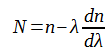for the difference between the group index ΔN applies: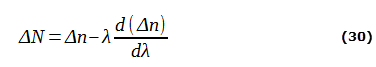and thereforeOn the other hand, equation (28) can be written as: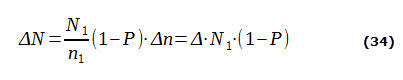which results, after comparison with equation (31), in the profile dispersion of the form:Thus, the profile dispersion can be determined by measuring the wavelength dependent numeric aperture (and therefore Δλ)).

#### Determination of the group delay of the individual eigenmodes

The group propagation delay of a certain eigenmode (l,p) per fiber length  τ = dβ/dω is a result of equation (15), if equation (15) is differentiated with respect to ω.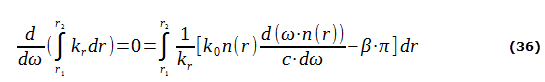In equation (36) the differentiation of kr with respect to ω (equation (8)) was used. The relation k0 = ω/c was also utilized. Equation (36) can be solved for the desired delay τ: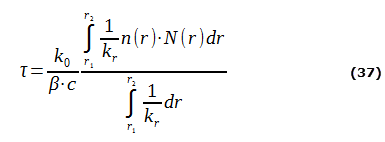with the group index N(r) = d(ω⋅n(r))/dω.

Firstly, for any arbitrary eigenmode (l,p), the phase constant β can be deteremined from equation (8) and equation (15). Then the group delay τ can be calculated from equation (37).

The refractive index profile n(r) (and therefore N(r), according to equation (28)), will now be optimized so that every eigenmode has the same propagation delay τ. In this general form, this is a complicated numerical optimization process.Figure 8: Potential profile for different profile exponents g

In this case, we limit ourselves on the profile class of the so-called potential profiles ("power-law-profiles") (see Figure 8), where the refractive index is described by: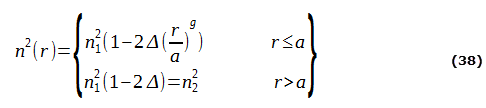with the profile exponent g.

For example the parabolic refractive index profile is described by g = 2 and the step-index profile is characterized with g = ∞.

For the refractive index profile according to equation (38) it applies that the propagation delay τ in equation (37) only depends on β, but doesn't depend on the circumferential order l (which is in principle contained in kr).

Under this condition, in analogy to equation (20) and (21), both the numerator and the denominator of equation (37) can be integrated over l without corruption of the result for τ.

After commutation of the integration order (shown in equation (21)) the integration over l can be solved analytically and takes the form: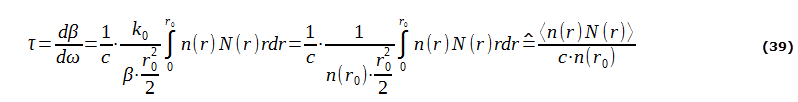where r0 is given by β = n(r0)/k0 (compare to Figure 9). The notation <...> in this context means the averaging over the fiber cross-section with r < r0.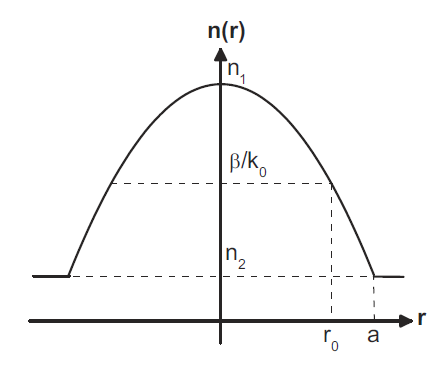Figure 9: Parabolic refraction index profile n(r) and the radius r0

In order to minimize the propagation delay differences between the eigenmodes, a profile optimization of the refractive index profile n(r) has to be determined. To achieve this aim the ration between the numerator and the denominator in equation (39) should be as independent as possible of r0 and β, respectively.

For a potential profile as shown in Figure 8 and linear profile dispersion (equation (28)):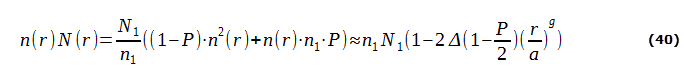applies. By using the approximation n(r0) ≈ n1(1-Δ(r0/a)g), equation (39) can be written as:#### Optimization of the refractive index profile

The propagation delay τ becomes independent with the approximation in equation (41) of r0 and therefore of β for the optimum profile exponent g = gopt, with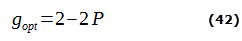As a result of the equation above without the approximations for n(r), a more accurate expression for the optimum profile exponent is given by:which differs only slightly from equation (42).

Since the profile dispersion parameter P is dependent of the material composition and the wavelength, also the optimum profile exponent gopt is dependent of these variables (see Figure 10 below).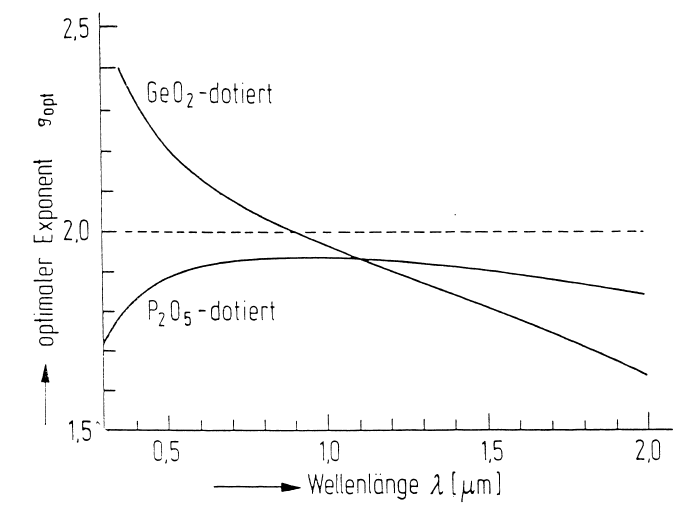Figure 10: Optimized profile exponent gopt for germanium and phosphorus doped silica glass as a function of the wavelength λ

For technology reasons mainly the GeO2-doping is used to diversify the refractive index. Thus, there are significant differences for the adjusting profile, whether the fiber should be employed for e.g. λ = 0.8µm or λ = 1.5µm. Due to the different delays of the individual modes a pulse broadening occurs, which limits the bandwidth. Hence the bandwidth is: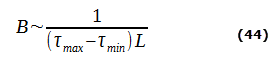τmax and τmin denote the minimum and maximum delay given by equation (41). from Figure 11 the maximum bandwidth of approximately 10GHz⋅km results for g = gopt. If the profile exponent g diverges from the optimal exponent gopt, according to Figure 11, the result is a minor bandwidth.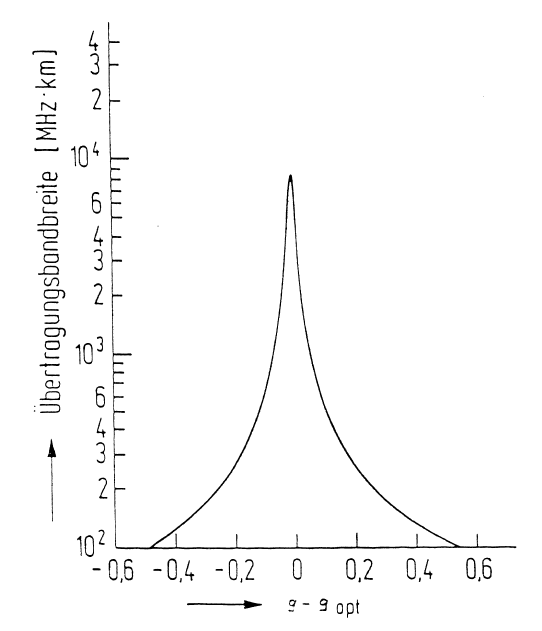Figure 11: 6 dB-Bandwidth of the transmitted electrical signal of a multi-mode graded-index with numerical aperture AN = 0, 24

In practice, the optical fiber manufacturing deviations |g-gopt| < 0.1 are manageable, so that bandwidths up to 1 GHz⋅km are still feasible.

In the consideration above, the bandwidth is inversely proportional to the fiber length. In fact, the bandwidth decreases less with increasing fiber length, perhaps in the formL0 is the fiber length to which the bandwidth B0 is related. This may due to:

1. The refractive index profile is subject to fluctuations, that means fiber pieces alternate between g - gopt > 0 and g - gopt < 0
2. According to micro curvature of the fiber, couplings occur between the waves. Hence, fast and slow waves exchange their energy with each other.

A typical dimension for multi-mode graded-index fibers would have, for example, a core diameter of 2a = 50µm, a cladding diameter of D = 125µm, a numerical aperture AN = 0.2 and a bandwidth of approximately 1GHz for 1km fiber length.